## How to calculate the sample time of MPC5744P ADC

cancel
Showing results for
Did you mean:
SOLVED

## How to calculate the sample time of MPC5744P ADC

838 ViewsContributor II

Dears,

Datasheet page 80 have a tab for ADC parameter: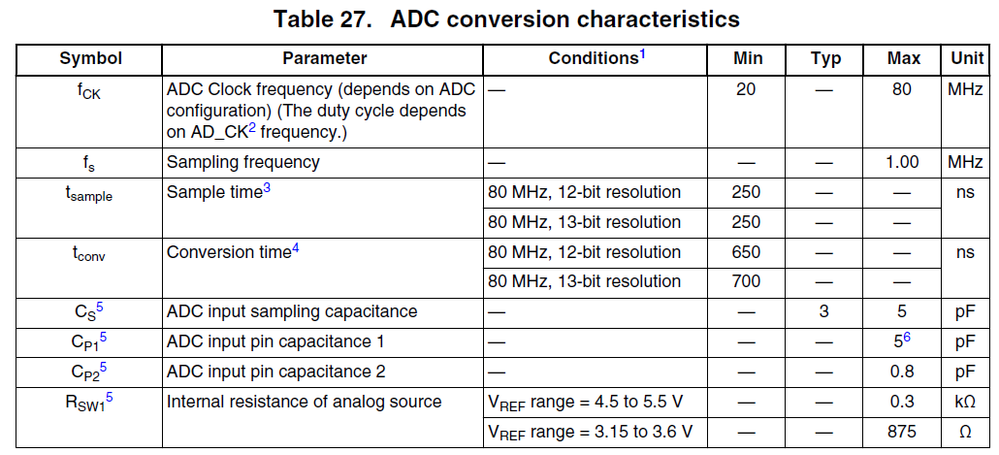Reference Manual page 1060 have a sequence chart: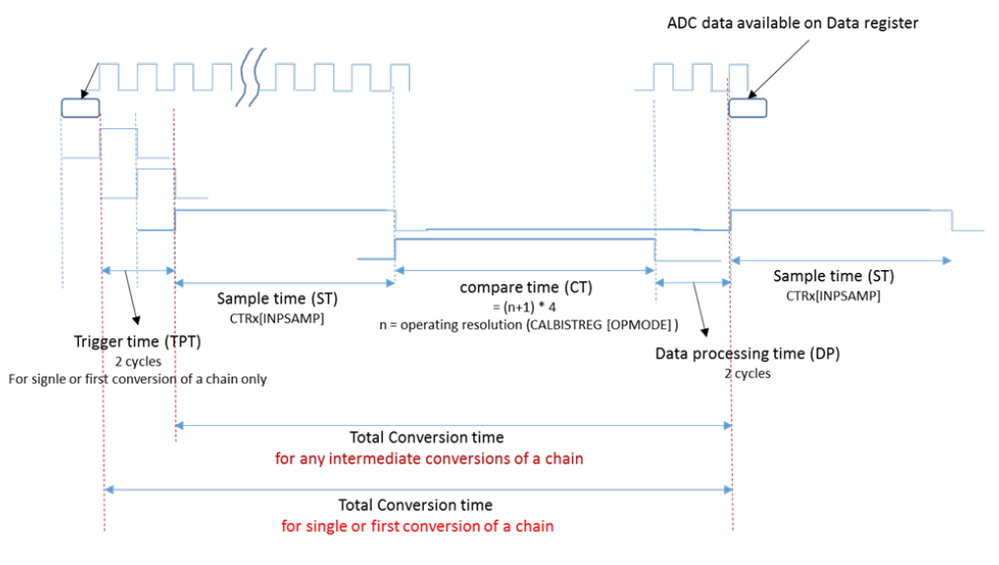I don't understand the meaning of Sample time and Conversion time, how to explain these?

Thanks.

Labels (1)
• ### General

Tags (3)
1 Solution
426 ViewsNXP TechSupport

3 Replies
426 ViewsNXP TechSupport

Hi, it is quite well described in RM section 36.5.11 Conversion time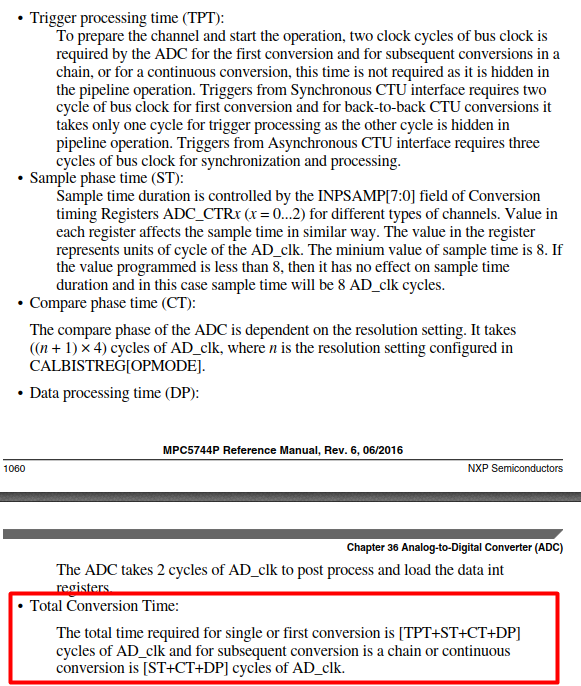In the following description there are examples for better understanding: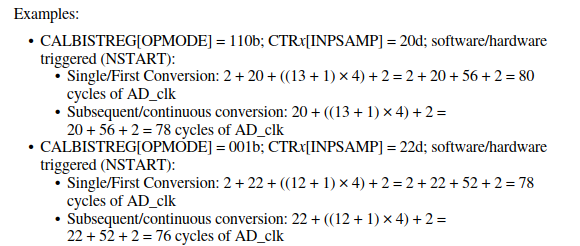Note that DS specifies conversion time and sample time individually (as mentioned in the note below the table) thus it is needed to sum both times to get total conversion time. In other words in this table conversion time means all phase excluding sample time.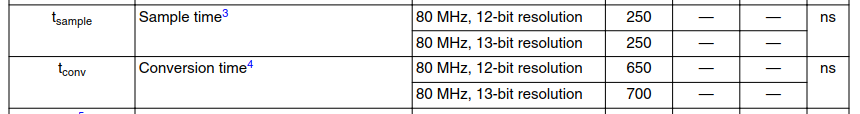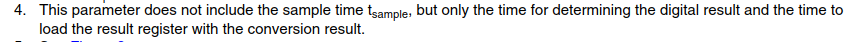426 ViewsContributor II

Hi David,

As your say, when ADC_CLK = 80MHz(per cycle equal to 12.5ns), sample resolution is 12-bit, Tsample MIN is 250ns, so MIN sample cycles is 20d, and Tconv MIN is 650ns(MIN covnv cycles is 52d), then get the following formula:

software/hardware triggered (NSTART):
Subsequent/continuous conversion: 20 + ((12 + 1) × 4) + 2 = 20 + 52 + 2 = 74 cycles of AD_clk

Tacd = 74 * 12.5 = 925ns, Fadc = 1s/925ns = 1.08MSPS > 1MSPS , right?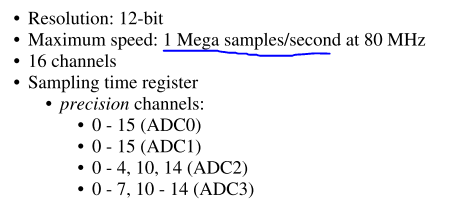Besides,

Why is there no ADC_CLK = 40MHz(Or other frequency) parameter for Tsample and Tconv in Table 27? because note that DS specifies "the minium value of sample time is 8".

427 ViewsNXP TechSupport